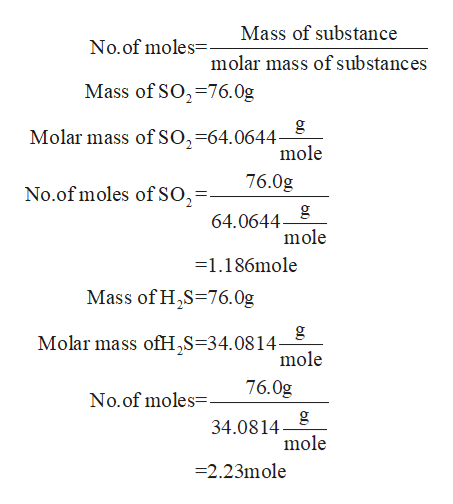# What is the maximum mass of S8 that can be produced by combining 76.0 g of each reactant?8SO2+16H2S⟶3S8+16H2Omass of S8 in grams:

Question
52 views

What is the maximum mass of S8 that can be produced by combining 76.0 g of each reactant?

8SO2+16H2S⟶3S8+16H2O

mass of S8 in grams:

check_circle

Step 1

Mass neither be created nor destroyed in a chemical reaction. Mass can be calculated in a balanced chemical equation by using law of conservation of mass.

Step 2

Firstly You calculate the no.of moles.help_outlineImage TranscriptioncloseMass of substance No.of moles-- molar mass of substances Mass of SO2-76.0g g Molar mass of SO,=64.0644 mole 76.0g No.of moles of SO2 11 g 64.0644 mole =1.186mole Mass of H2S 76.0g g Molar mass ofHS=34.0814- mole 76.0g No.of moles 34.0814 mole =2.23mole fullscreen
Step 3

From the equation 1 moles of sulfur dio...

### Want to see the full answer?

See Solution

#### Want to see this answer and more?

Solutions are written by subject experts who are available 24/7. Questions are typically answered within 1 hour.*

See Solution
*Response times may vary by subject and question.
Tagged in

### Quantitative analysis